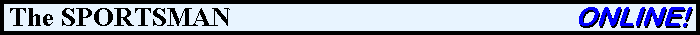Articles Documents Equipment Events Links Membership Miscellaneous Scrapbook Targets What's New

Calculate Kinetic EnergyThe general formula for Kinetic Energy is

KE = ½ x Mass x Velocity²

If we calculate through all the conversion factors when using the units of
measure commonly used in the field of sporting ballistics, the formula becomes:where V is the velocity of the bullet in feet per second,
and WB is the weight of the bullet in grains.

Kinetic Energy Calculator

Back to the Miscellaneous Menu